### Choose language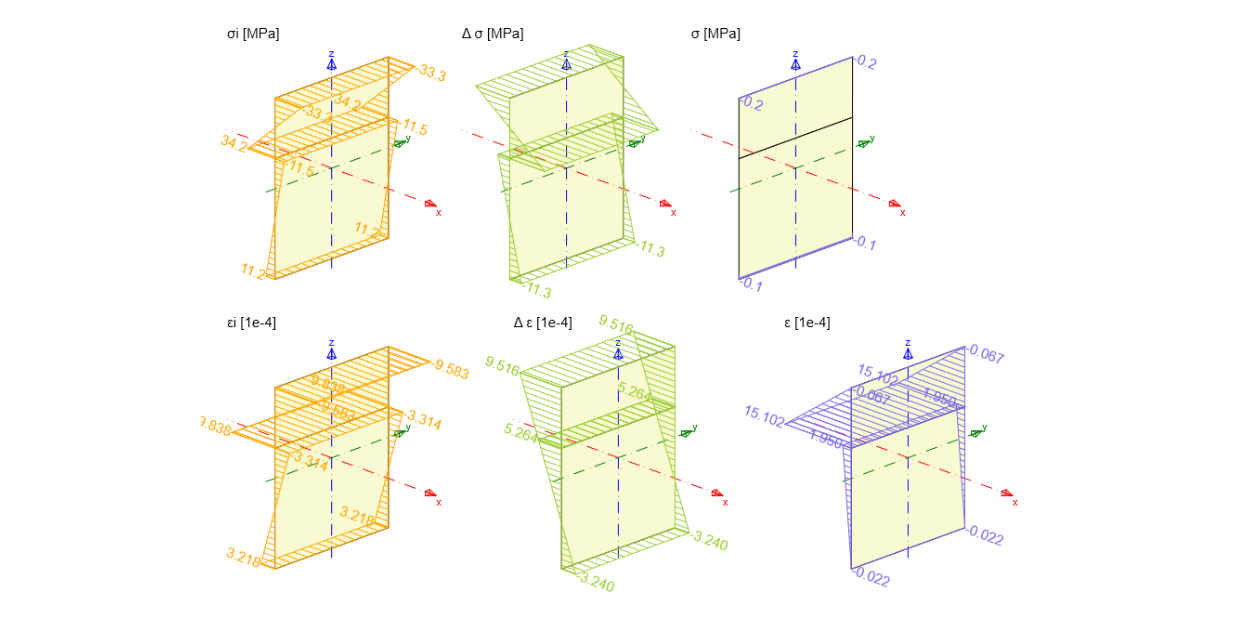# Plane of strains on the composite section

Let's have a look at the workflow of the recalculation of internal forces on composite cross-section (obtained from FEM software) to plane of strains, which can be inserted into RCS.
$$Cross-section Concrete grade C30/37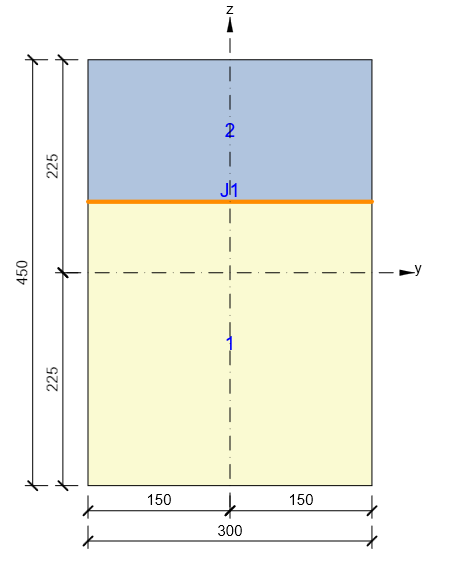Construction stages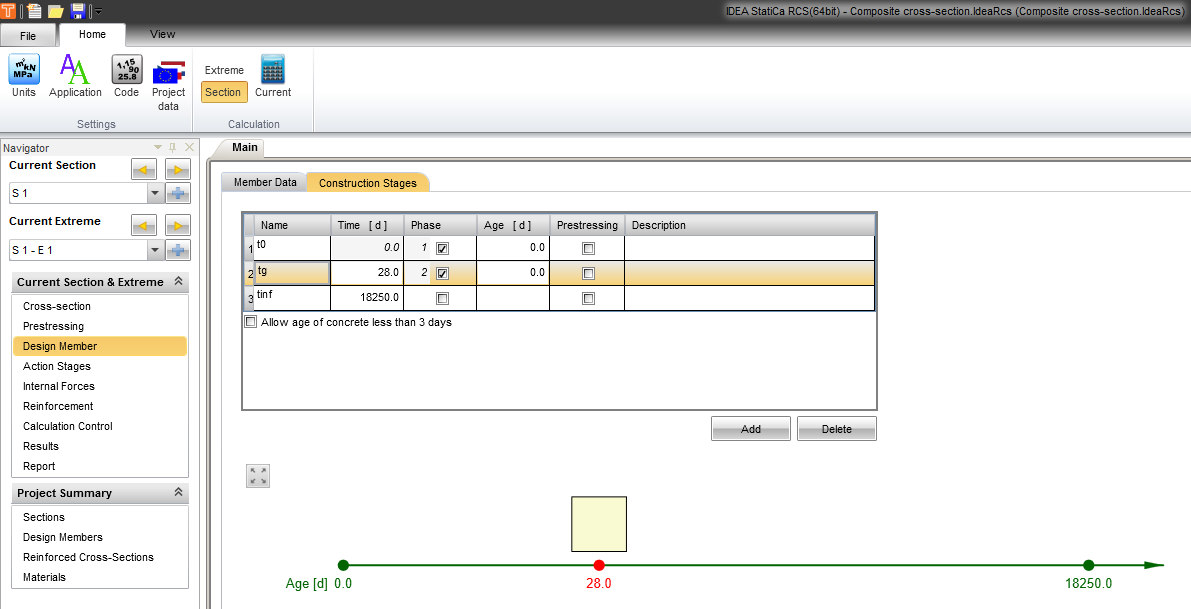Internal forces Internal forces received from the third-party software for each part of the section separately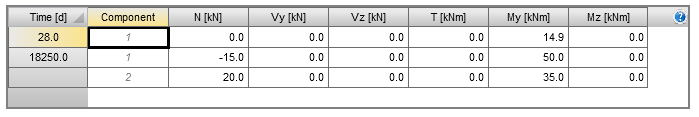Plane of the strain at 28 days for component 1 Tangent modulus of elasticity is used for calculation. The tangent modulus is equaled to 1.05 · $$E_{cm}$$. Internal forces are recalculated to the center of gravity of whole cross-section (with concrete slab)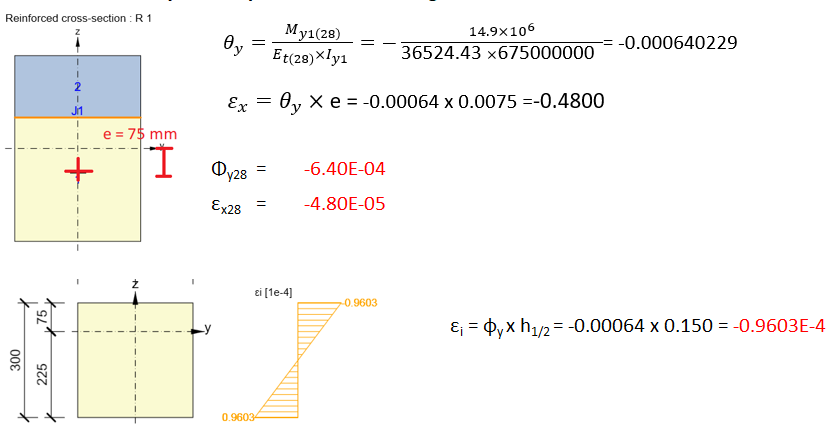Plane of the strain at 18,250 days for component 1 - prefabricated part The curvature is recalculated to the center of gravity of the total cross-section (with a concrete slab). The normal force acting in the center of gravity of the lower (prefabricated) part is located eccentrically to the center of gravity of the total cross-section (with a concrete slab) and causes an additional bending moment. An axial strain $$ε_{x1}$$ at the center of gravity of the total cross-section (with concrete slab) defines the displacement of the plane of the strain calculated on the prefabricated part relative to the center of gravity of the total cross-section (with concrete slab).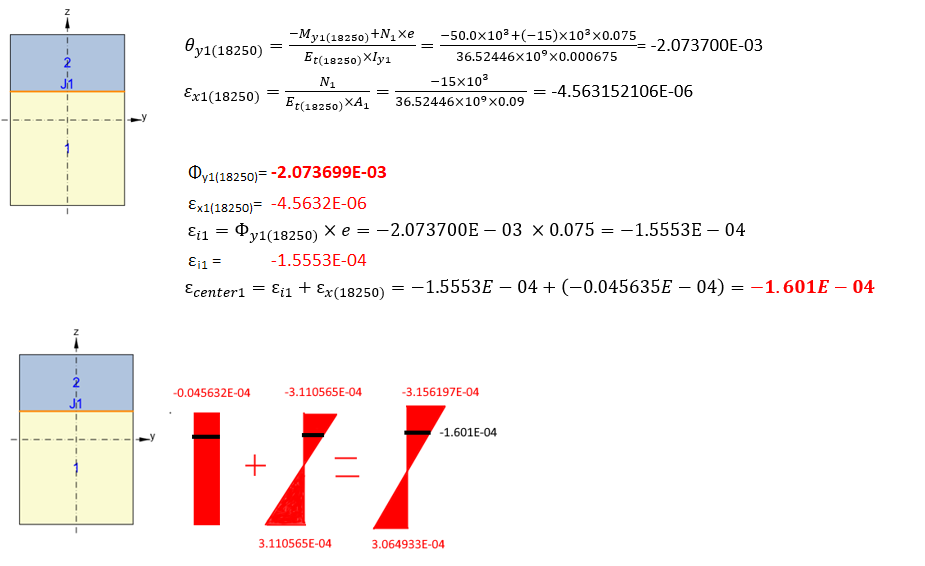Plane of the strain at 18,250 days for component 2 - composite slab The curvature is recalculated to the center of gravity of the total cross-section (with a concrete slab). The normal force acting in the center of gravity of the upper part (concrete slab) is located eccentrically to the center of gravity of the total cross-section (with a concrete slab) and causes an additional bending moment. An axial strain $$ε_{x2}$$ at the center of gravity of the total cross-section (with concrete slab) defines the displacement of the plane of the strain calculated on the prefabricated part relative to the center of gravity of the total cross-section (with concrete slab).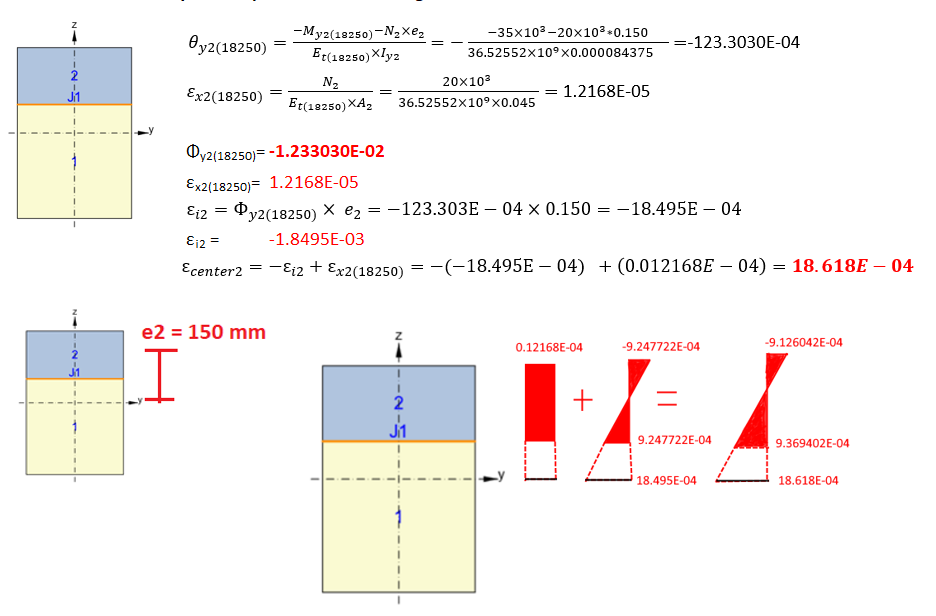Calculated plane of strains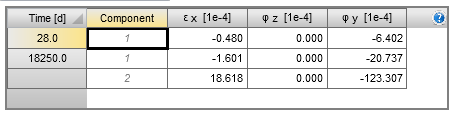Attached Downloads Plane of strains on the composite section.zip (ZIP, 60 kB)$$